Intuitionistic Logic Explorer < Previous   Next > Nearby theorems Mirrors  >  Home  >  ILE Home  >  Th. List  >  r19.21be Unicode version

Theorem r19.21be 2453
 Description: Inference from Theorem 19.21 of [Margaris] p. 90. (Restricted quantifier version.) (Contributed by NM, 21-Nov-1994.)
Hypothesis
Ref Expression
r19.21be.1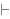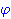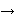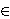Assertion
Ref Expression
r19.21beProof of Theorem r19.21be
StepHypRef Expression
1 r19.21be.1 . . . 421r19.21bi 2450 . . 3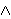32expcom 114 . 243rgen 2417 1Colors of variables: wff set class Syntax hints:wi 4wcel 1434wral 2349 This theorem was proved from axioms:  ax-1 5  ax-2 6  ax-mp 7  ax-ia1 104  ax-ia2 105  ax-ia3 106  ax-gen 1379  ax-4 1441 This theorem depends on definitions:  df-bi 115  df-ral 2354 This theorem is referenced by: (None)
 Copyright terms: Public domain W3C validator